# KSEEB SSLC Class 10 Maths Solutions Chapter 9 Polynomials Ex 9.2

KSEEB SSLC Class 10 Maths Solutions Chapter 9 Polynomials Ex 9.2 are part of KSEEB SSLC Class 10 Maths Solutions. Here we have given Karnataka SSLC Class 10 Maths Solutions Chapter 9 Polynomials Exercise 9.1.

## Karnataka SSLC Class 10 Maths Solutions Chapter 9 Polynomials Exercise 9.2

Question 1.
Find the zeroes of the following quadratic polynomials and verify the relationship between the zeroes and their coefficients
(i) x2 – 2x – 8
(ii) 4s2 – 4s + 1
(iii) 6x2 – 3 – 7x
(iv) 4u2 – 8u
(v) t2 – 15
(vi) 3x2 – x – 4
Solution: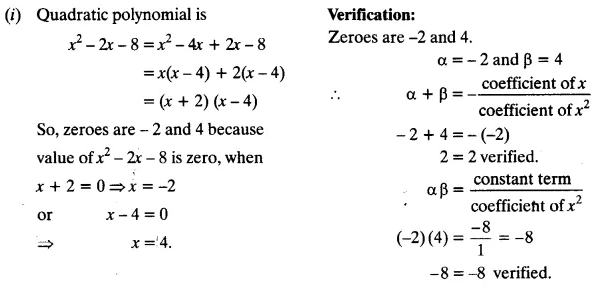(iv) 4u2 – 8u
= 4u2 – 8u + 0
= 4u (u – 2)
If 4u = 0, then u = 0
If u – 2 = 0, then u = 2
∴ Zeroes are 0 and 2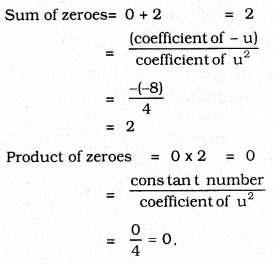(v) t2 – 15
= t2 + 0 – 15(vi) 3x2 – x – 4
= 3x2 – 4x + 3x – 4
= x(3x – 4) + 1 (3x – 4)
= (3x – 4) (x + 1)
If 3x – 4 = 0, then x = $$\frac{4}{3}$$
If x + 1 = 0, then x = -1
∴ Zeroes are $$\frac{4}{3}$$ and -1.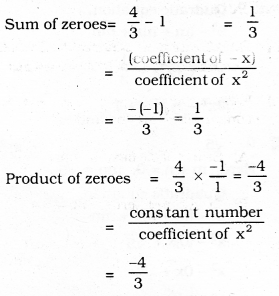Question 2.
Find a quadratic polynomial each with the given numbers as the sum and product of its zeroes respectively.
(i) $$\frac{1}{4}$$, 1
(ii) $$\sqrt{2}, \frac{1}{3}$$
(iii) 0, $$\sqrt{5}$$
(iv) 1, 1
(v) $$-\frac{1}{4}, \frac{1}{4}$$
(vi) 4, 1
Solution:
(i) $$\frac{1}{4}$$, 1
Here m + n = $$\frac{1}{4}$$, mn = -1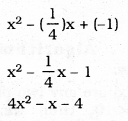(ii) $$\sqrt{2}, \frac{1}{3}$$
Here m + n = $$\sqrt{2}$$, mn = $$\frac{1}{3}$$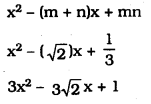(iii) 0, $$\sqrt{5}$$
Here m + n = 0, mn = $$\sqrt{5}$$(iv) Standard form of quadratic polynomial
sum and product of its zeroes is
K(x2 – sum of the zeroes) x + product of zeroes.
= K(x2 – 1x + 1)
Taking K = 1
= x2 – x + 1

(v) $$-\frac{1}{4}, \frac{1}{4}$$
Here m + n = $$-\frac{1}{4}$$, mn = $$\frac{1}{4}$$